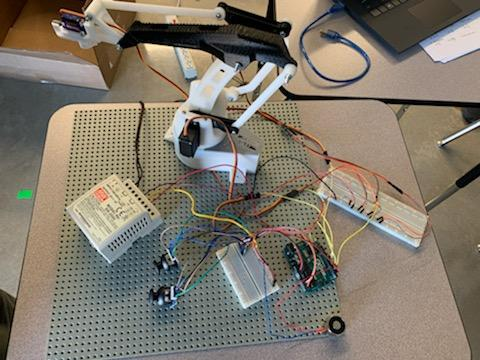# Problem with overheating with robotic arm

I am writing this for my student who has been working independently on an Arduino project found here: https://www.instructables.com/Joystick-Controlled-Robot-Arm-Using-an-Arduino/
I will provide the picture I took of his project below.

Not being very experienced in arduino beyond basic tutorials, here is what I am wondering. It is overheating potentially because the servos work on 3 amps and there are 4 of them in series so this seems like way to much to handle. Additionally, in the video, it looks like there is another component that the servos and power are connected, but I do not see this in the schematics or item list (it is the portion directly next to the base of the robotic arm in the video). I have more images I can share but am reduced to 1 since a new user I amWhat is overheated?

Servos have to be powered independently of the Arduino (be sure to connect the grounds), and the power supply must be capable of handling the maximum current draw of all servos working together.

Also, breadboards cannot handle motor currents, so the servo wiring cannot be routed through the breadboard. The breadboard tracks quickly burn out if you try.

We generally recommend to avoid Instructables, as most of them are crap, written by people who do not understand what they are doing. This one is not an exception.

1 Like

Thank you! I will advise against instructables in the future and will search here for similar projects so that we might learn how to address this problem

MG996 55g Servo Specifications:

• Working current: 100mA

ref: eBay

Q: What is overheating

A: The breadboard directly where the power supply enter, and the first wire (the servo wire) directly after that power input wire. In the picture it is the breadboard to the right.

Post a wiring diagram, and code.

I would always do something like this to be safe. They could be the same supply, just as long as the servo power doesn’t come from the Arduino.

• Running Current 500mA - 900mA @ 6V
• Stall Current 2.5A @ 6V

NOT eBay

2 Likes

Exactly. The breadboard tracks are burned. You should solder all the power connections together, or twist bare wires together and secure the connection with screw terminal blocks rated for 10 Amperes.

I'm guessing that the construction shown in the Instructable was abandoned shortly after the "lesson" was posted.

1 Like

Sorry, they were in the link since ?I did not think I could post more, but I see I can post in replies.

and here is the code:

#include <Servo.h>

// Create servo objects
Servo servo1;
Servo servo2;
Servo servo3;
Servo servo4;

int pin0 = A0;
int pin1 = A1;
int pin2 = A2;
int pin3 = A3;

// Setup of angle variables we have to use further down in the program
int angle1;
int angle2;
int angle3;
int angle4;

// Setup of value variables we have to use further down in the program
int value1;
int value2;
int value3;
int value4;

// Setup of limit values
long int forservo1 = 20000;
long int forservo2 = 20000;
long int forservo3 = 20000;
long int forservo4 = 20000;

void setup()
{
pinMode(3, INPUT); /* Use button on thumbstick to set pin 3 as input /
pinMode(4, OUTPUT); /
Set pin 4 as output to the magnet */

// Attaches the servo on pins to the servo objects
servo1.attach(5);
servo2.attach(6);
servo3.attach(9);
servo4.attach(10);
}

void loop()
{
// read analog values from joysticks

// 10 bit value is too big, so we change the scale from 0 - 1023 proportionately to 1 - 29
value1 = map(value1, 0, 1023, 1, 29);
value2 = map(value2, 0, 1023, 1, 29);
value3 = map(value3, 0, 1023, 1, 29);
value4 = map(value4, 0, 1023, 1, 29);

// it needs correction of positions, because joysticks are not very precise to stay exactly in the center
if(value1 <= 17 && value1 >= 13) // if value1 if between 13-17, then it's equal to 15 */
value1 = 15;
if(value2 <= 17 && value2 >= 13)
value2 = 15;
if(value3 <= 17 && value3 >= 13)
value3 = 15;
if(value4 <= 17 && value4 >= 13)
value4 = 15;

// Change initial value 'forservo' which is used to turn servo slower. This value has got very big scale.
// We add or substract (depends on turning direction) difference between analog value and center from 'forservo'
forservo1 = forservo1 - (value1 - 15);
forservo2 = forservo2 - (value2 - 15);
forservo3 = forservo3 + (value3 - 15);
forservo4 = forservo4 + (value4 - 15);

// this part do not let variables 'forservo' go out of the limits
if(forservo1 < 1) // if forservo1 is less than 1, then it's equal to 1
forservo1 = 1;
if(forservo1 > 20000) // if forservo1 is greater than 20000, then it's equal to 20000
forservo1 = 20000;
if(forservo2 < 1)
forservo2 = 1;
if(forservo2 > 20000)
forservo2 = 20000;
if(forservo3 < 1)
forservo3 = 1;
if(forservo3 > 20000)
forservo3 = 20000;
if(forservo4 < 1)
forservo4 = 1;
if(forservo4 > 20000)
forservo4 = 20000;

// change used value to angle of servo. Angles are adjusted during testing
angle1 = map(forservo1, 1, 20000, 10, 180);
angle2 = map(forservo2, 1, 20000, 10, 180);
angle3 = map(forservo3, 1, 20000, 10, 180);
angle4 = map(forservo4, 1, 20000, 10, 180);

// send angles to servos
servo1.write(angle1);
servo2.write(angle2);
servo3.write(angle3);
servo4.write(angle4);

// activates the magnet
while (digitalRead(3) == HIGH) { /* Read pin 3 from the thumbstick /
digitalWrite(4, HIGH); /
Write to pin 4 (magnet) */
}

}

This topic was automatically closed 120 days after the last reply. New replies are no longer allowed.